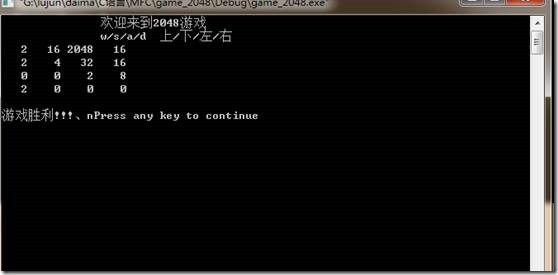# STM32实现2048游戏（一） C实现

0

先在PC上，用c语言实现2048游戏。用的软件是vc6.0，比较老的软件了。

2048游戏用c语言实现，其实也还是比较容易的，本质上就是一个二维数组，每次通过输入的值，判断是上移，下移，左移，右移后，再去改变这个二维数组的值，然后将二维数组的值显示出来就行了。

```           int b = { 16,8,0,8,
16,1024,0,8,
4,32,0,8,
2,0,0,8};

void zuoyi(int a);
void youyi(int a);
void shangyi(int row);
void xiayi(int row);
int  iswin();
int isfailure();

void display();
void gennew_pane();```

定义的b就是所需要的二维数组，可以预先往数组里面写入值。然后就是几个函数：

zuoyi(int a)                    : 左移

youyi(int a)                  ：右移

shangyi(int row)                上移

xiayi(int row)                      下移

iswin()                               判断是否胜利

isfailure()                          ：判断是否失败

display()                             : 对二位数组显示

gennew_pane()   ：产生新的值

```int main(int argc, char* argv[])
{
char c;
display();
while(1)
{
scanf("%c",&c);
fflush(stdin);
if( c == 'a' )
{
zuoyi(b);
zuoyi(b);
zuoyi(b);
zuoyi(b);
gennew_pane();
system("cls");
display();
if(isfailure())
{
printf("游戏失败\n");
break;
}
}
else if( c== 'd')
{
youyi(b);
youyi(b);
youyi(b);
youyi(b);
gennew_pane();
system("cls");
display();
if(isfailure())
{
printf("游戏失败\n");
break;
}
}
else if( c== 'w')
{
shangyi(0);
shangyi(1);
shangyi(2);
shangyi(3);
gennew_pane();
system("cls");
display();
if(isfailure())
{
printf("游戏失败\n");
break;
}
}
else if( c=='s')
{
xiayi(0);
xiayi(1);
xiayi(2);
xiayi(3);
gennew_pane();
system("cls");
display();
if(isfailure())
{
printf("游戏失败\n");
break;
}
}
else
{
printf("输入错误，请重新输入\n");
}
if(iswin())
{
printf("游戏胜利!!!、n");
break;
}

}

return 0;
}```

主函数其实做的事很简单，就是读取输入的值，判断是往那一边移位，然后再对数组进行操作，再将二维数组给打印出来，判断是否游戏胜利或者失败，胜利就打印出胜利，失败就打印失败，如果没有胜利或失败，就在等待输入，进行下一轮。

下面就是实现各个函数

1、  左移，参数是行数组，先对值一样的合并，再往左边移动，保证非零的值永远在左边。

```void zuoyi(int a)
{
int i;
int j;
//merge
for(i=0; i<3; i++)
{

if(a[i] == a[i+1])
{
a[i] = a[i] + a[i+1];
a[i+1] = 0;
}

}
//zuoyiwei
for(i=0; i<3; i++)
{
if(a[i] == 0)
{
for(j=i; j<3; j++)
a[j] = a[j+1];
a[j] = 0;
}
}
}```

2、  右移，参数是行数组，先对值一样的合并，再往右边移动，保证非零的值永远在右边。

```void youyi(int a)
{
int i;
int j;
//merge
for(i=3; i>0; i--)
{

if(a[i] == a[i-1])
{
a[i] = a[i] + a[i-1];
a[i-1] = 0;
}

}
//youyiwei
for(i=3; i>0; i--)
{
if(a[i] == 0)
{
for(j=i; j>0; j--)
a[j] = a[j-1];
a[j] = 0;
}
}
}```

3、  上移，输入参数是第几列。将列的值一样的合并，在往上移，保证非零的数永远在上

```void shangyi(int column)
{
int i;
int j;
//merge
for(i=0; i<3; i++)
{

if(b[i][column] == b[i+1][column])
{
b[i][column] = b[i][column] + b[i+1][column];
b[i+1][column] = 0;
}

}
//shangyiwei
for(i=0; i<3; i++)
{
if(b[i][ column] == 0)
{
for(j=i; j<3; j++)
b[j][column] = b[j+1][column];
b[j][column] = 0;
}
}
}```

4、  下移，输入参数是第几列。将列的值一样的合并，在往下移，保证非零的数永远在下

```void xiayi(int column)
{
int i;
int j;
//merge
for(i=3; i>0; i--)
{
if(b[i][column] == b[i-1][column])
{
b[i][column] = b[i][column] + b[i-1][column];
b[i-1][column] = 0;
}
}
//xiayiwei
for(i=3; i>0; i--)
{
if(b[i][ column] == 0)
{
for(j=i; j>0; j--)
b[j][column] = b[j-1][column];
b[j][column] = 0;
}
}
}```

5、  判断赢，这个就依次检查二维数组是否有一个值是2048就行了。

```int iswin()
{
int i;
int j;
for(i=0; i<4; i++)
for(j=0; j<4; j++)
if(b[i][j] == 2048)
return 1;
return 0;
}```

6、  判断输，这个要相对麻烦点。要输的话，首先数组中，没有一个值是0，其次，没有一个值和他的上下左右是一样的，也就是下一次不能移动了，这就输了。在这里，是这样判断的，遍历前三行前三列的每一个元素，如果以该元素为左上角的4方块数据有一个为0，说明还可以移动，游戏还没有结束。如果该元素和右边的数据，或者下边的数据一样的话，说明可以移动，游戏也还没有结束。如果该元素的下边数据和该元素的右下角数据一样的话，或者该元素的右边数据和该元素的有下家数据一样的话，说明可以移动，游戏也还没有结束。除了上面的所有情况下，说明移动不了，认定游戏失败

```int isfailure()
{
int i;
int j;
//three row
for(i=0; i<3; i++)
{
for(j=0; j<3; j++)
{
if(b[i][j] == 0 || b[i][j+1] == 0 || b[i+1][j] == 0 || b[i+1][j+1] == 0)
return 0;
if(b[i][j] == b[i][j+1])
return 0;
if(b[i][j] == b[i+1][j])
return 0;
if(b[i+1][j] == b[i+1][j+1])
return 0;
if(b[i][j+1] == b[i+1][j+1])
return 0;
}
}
return 1;
}```

7、  产生新的值。在每次移动后，要随机产生一个新的2值。这个就比较容易了，使用随机函数，得到坐标，判断坐标处值是否为0，为0，说明可以在该地方产生。

```void gennew_pane()
{
int i;
int j;
int k=0;
srand(time(0));
while(1)
{
i=rand()%4;
j=rand()%4;
if(b[i][j] == 0)
{
b[i][j] = 2;
break;
}
k++;
if(k>200)
break;
}
}```

8、  打印，就是将二维数组给打印出来，打印出来的效果和2048游戏是一样的，只是没有图形化界面而已

```void display()
{
int i;
int j;
printf("               欢迎来到2048游戏\n");
printf("               w/s/a/d  上/下/左/右\n");
for(i=0; i<4; i++)
{
for(j=0; j<4; j++)
printf("%4d ",b[i][j]);
printf("\n");
}
printf("\n");
}```以上，就用c语言实现了2048游戏了。后面要将这个游戏给移植到STM32上面去，用TFT屏显示界面。

关于上面所说的bug，各位神仙大神，可是有什么好的方法解决吗？